Tamilnadu State Board New Syllabus Samacheer Kalvi 9th Science Guide Pdf Chapter 13 Chemical Bonding Text Book Back Questions and Answers, Notes.

## Samacheer Kalvi 9th Science Solutions Chapter 13 Chemical Bonding

### 9th Science Guide Chemical Bonding Text Book Back Questions and Answers

Question 1.
Number of valence electrons in carbon is
(a) 2
(b) 4
(c) 3
(d) 5
(b) 4Question 2.
Sodium having atomic number 11, is ready to ______________ electron/electrons to attain the nearest noble gas electronic configuration.
(a) gain one
(b) gain two
(c) lose one
(d) lose two
(c) lose one

Question 3.
The element that would form anion by gaining electrons in a chemical reaction is ………………..
(a) potassium
(b) calcium
(e) fluorine
(d) iron
(c) fluorineQuestion 4.
Bond formed between a metal and non metal atom is usually …………………..
(a) ionic bond
(b) covalent bond
(e) co-ordinate bond
(a) ionic bond

Question 5.
______________ compounds have high melting and boiling points.
(a) Covalent
(b) Coordinate
(e) Ionic
(c) IonicQuestion 6.
Covalent bond is formed by …………………
(a) transfer of electrons
(b) sharing of electrons
(c) sharing a pair of electrons
(b) sharing of electrons

Question 7.
Oxidising agents are also called as …………………. because they remove eletrons form other substances.
(a) electron donors
(b) electron acceptors
(b) electron acceptorsQuestion 8.
Elements with stable electronic configurations have eight electrons in their valence shell. They are ……………….
(a) halogens
(b) metals
(c) nobel gases
(d) non metals
(c) noble gases

Question 1.
How do atoms attain Noble gas electronic configuration?
Atoms of all elements, other than inert gases, combine to form molecules because they have incomplete valence shell and tend to attain a stable electronic configuration similar to noble gases. Atoms can combine either by transfer of valence electrons from one atom to another or by sharing of valence electrons in order to achieve the stable outer shell of eight electrons.Question 2.
NaCl is insoluble in carbon tetrachloride but soluble in water. Give reason.
NaCl is an ionic compound, it is soluble in polar solvent (water). Whereas CCl4 is a covalent compound. So.it is insoluble in polar solvent (water). But it is soluble in nonpolar solvents.

Question 3.
Explain the Octet rule with an example.
The tendency of atoms to have eight electrons in the valence shell is known as the ‘Octet rule’ or the ‘Rule of eight’ For example, Sodium with atomic number 11 will readily loose one electron to attain Neon’s stable electronic configuration. Similarly, chlorine has electronic configuration 2,8,7. To get the nearest noble gas (i.e. Argon) configuration, it needs one more electron. So chlorine readily gains one electron from another atom and obtains stable electronic configuration. Thus elements tend to have stable valence shell (eight electrons) either by losing or gaining electrons.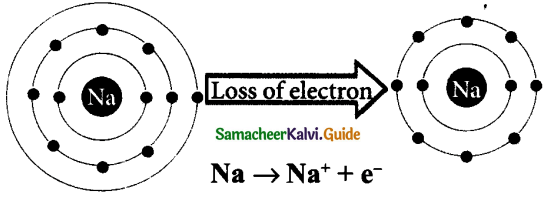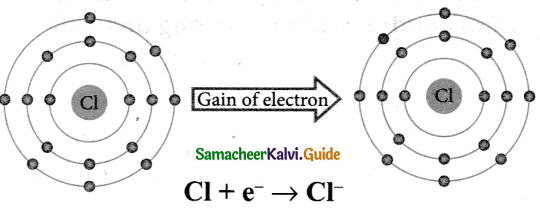Question 4.
Write a note on different types of bonds.
There are different types of chemical bonding possible between atoms which make the molecules. Depending on the type of bond they show different characteristics or properties.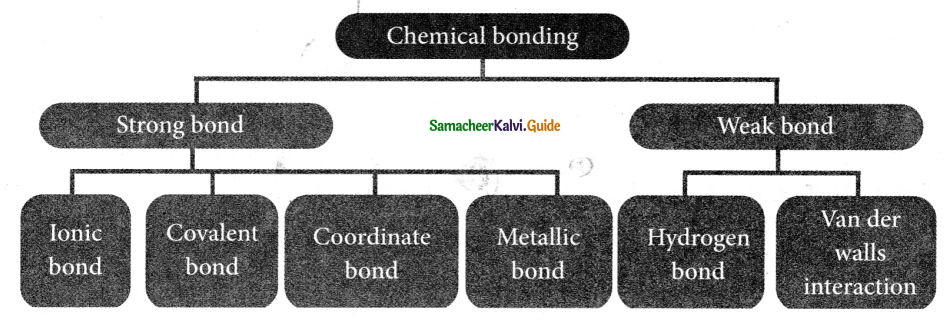Question 5.
Correct the wrong statements.
(a) Ionic compounds dissolve in non-polar solvents.
(b) Covalent compounds conduct electricity in molten or solution state.
(a) Covalent compounds dissolve in non-polar solvents. (or) Ionic compounds dissolve in polar solvents.
(b) Ionic compounds conduct electricity in molten or solution state.Question 6.
Complete the table given below.Question 7.
Draw the electron distribution diagram for the formation of Carbon dioxide (CO2) molecule.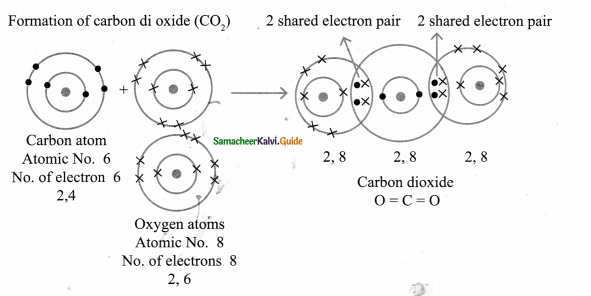Question 8.
Fill in the following table according to the type of bonds formed in the given molecule.
CaCl2, H2O, CaO, CO, KBr, HCl, CCl4, HF, CO2, Al2Cl6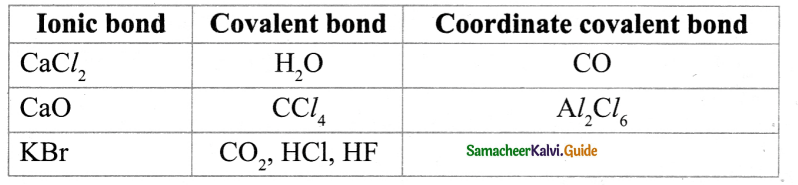Question 9.
The property which is characteristic of an Ionic compound is that
(a) it often exists as a gas at room temperature.
(b) it is hard and brittle.
(c) it undergoes molecular reactions
(d) it has a low melting point.
(b) it is hard and brittleQuestion 10.
Identify the following reactions as oxidation or reduction.
(a) Na → Na+ + e
(b) Fe3+ + 2 e → Fe+
(a) oxidation
(b) reduction

Question 11.
Identify the compounds as Ionic/Covalent/Coordinate based on the given characteristics.
(a) Soluble in non-polar solvents
(b) Undergoes faster/instantaneous reactions
(c) Non-conductors of electricity
(d) Solids at room temperature
(a) Co-ordinate Covalent compound.
(b) Ionic compound.
(c) Covalent compound.
(d) Ionic compound.Question 12.
An atom X with atomic number 20 combines with atom Y with atomic number 8. Draw the dot structure for the formation of the molecule XY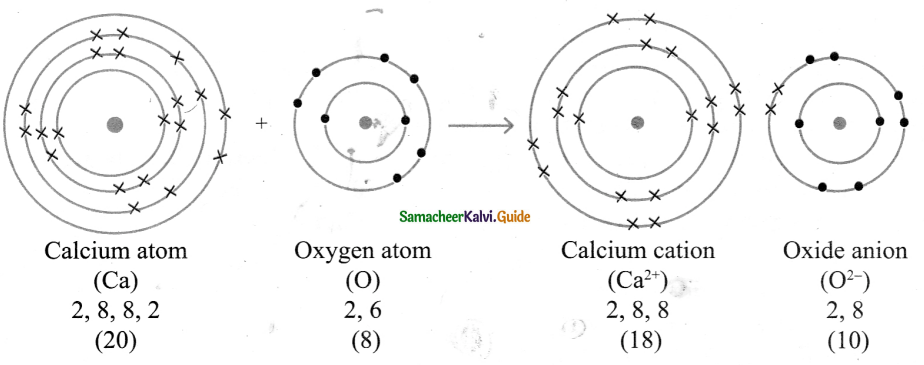Question 13.
Considering MgCl2 as ionic compound and CH4 as covalent compound give any two differences between these two compounds.

 MgCl (ionic compound) CH4(covalent compound) 1. It is formed by the transfer of electrons (2e– ) from metal magnesium (Mg) to a non – metal atom chlorine (Cl) It is formed by the sharing of electrons between the non-metal atoms carbon and hydrogen 2. In MgCl2 , strong electrostatic force of attraction exist between magnesium cation (Mg2+) and chlorine atoms (Cl– ) In CH4, weak force of attraction exist between the carbon atom and four hydrogen atoms.Question 14.
Why are Noble gases inert in nature?
Noble gases are inert in nature due to the completely filled subshells and thus have stable electronic structures which is very difficult to change. The elements Helium, Neon, Argon, Krypton, Xenon and Radon of group 18 in the periodic table are Noble gases.

Question 1.
List down the differences between Ionic and Covalent compounds.

 Ionic Compounds Covalent Compounds Formed by the transfer of electrons from a metal to a non-metal atom Formed by sharing of electrons between non-metal atoms. The strong electrostatic force of attraction between cations and anions. Mutual sharing of electrons and so weak force of attraction between atoms. Solids at room temperature. Gases, liquids, and soft solids. Conducts electricity in molten state or in solutions Non-conductors of electricity. Have high melting and boiling points. Have low melting and boiling points. Soluble in polar solvents Soluble in non-polar solvents. Hard and brittle. Soft and waxy. Undergo ionic reaction which is fast and instantaneous Undergo molecular reactions which are slow.Question 2.
Give an example for each of the following statements.
(a) A compound in which two Covalent bonds are formed.
(b) A compound in which one ionic bond is formed.
(c) A compound in which two Covalent and one Coordinate bond are formed.
(d) A Compound in which three covalent bonds are formed.
(e) A compound in which a coordinate bond is formed.
(a) Oxygen molecule (O2) (O = O)
(b) Sodium’Chloride (NaCl)
(c) Carbon monoxide?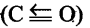(d) Nitrogen molecule (N2) (N ≡N)
(e) NH3 → BF3

Question 3.
Identify the incorrect statement and correct them.
(a) Like covalent compounds, coordinate compounds also contain charged particles (ions). So they; are good conductors of electricity.
(b) Ionic bond is a weak bond when compared to Hydrogen bond.
(c) Ionic or electrovalent bonds are formed by the mutual sharing of electrons between atoms.
(d) Loss of electrons is called Oxidation and gain of the electron is called Reduction.
(e) The electrons which are not involved in bonding are called valence electrons.
(a) Incorrect statement. Like covalent compounds, co-ordinate compounds also do not contain charged particles (ions), so they are bad conductors of electricity.
(b) Incorrect statement. An ionic bond is a strong bond when compared to a hydrogen bond.
(c) Incorrect statement. Covalent bonds are formed by the mutual sharing of electrons between atoms, (or) Ionic or electrovalent bonds are formed by the transfer of electrons between atoms.
(d) Correct statement
(e) Incorrect statement. The electrons which are not involved in bonding are called lone pair of electrons.Question 4.
Discuss in brief the properties of coordinate covalent compounds.
The compounds containing coordinate covalent bonds are called coordinate compounds.
(a) Physical state – These compounds exist as gases, liquids or solids.
(b) Electrical conductivity-Like covalent compounds, co-ordinate compounds also do not contain charged particles (ions), so they are bad conductors of electricity.
(c) Melting point – These compounds have to melt and boiling points higher than those of purely covalent compounds but lower than those of purely ionic compounds.
(d) Solubility – Insoluble in polar solvents like water but are soluble in non-polar solvents like benzene, CCl4, and toluene.
(e) Reactions – Co-ordinate covalent compounds undergo molecular reactions which are slow.

Question 5.
Find the oxidation number of the elements in the following compounds.
(a) C in CO2
(b) Mn in MnSO4
(c) N in HNO3
(a) C in CO2
1(C) + 2(0) = 0
1x + 2(-2) = 0
x-4 = 0 ‘
x = +4 .
ON of C in CO2 is +4

(b) Mn in MnSO4
1 (Mn) + 1 (S) + 4(0) = 0
x + 1(+6) + 4(-2) = 0
x + 6 – 8 = 0
x – 2 = 0
x = +2
ON of Mn in MnSO4 is +2,

(c) N in HNO3
1(H) + 1 (N) +3(0) =0
1 (+1) + 1 (x) + 3 (-2) =0
+ 1 + x – 6 =0
x – 5 =0
x = +5
ON of N in HNO3 is + 5.Question 1.
Which of the following atom can exist independently?
(a) Magnesium
(b) Chlorine
(c) Hydrogen
(d) Neon
(d) Neon

Question 2.
Alkali and alkaline earth metals form ………… compound when they react with non-metals.
(a) ionic
(b) covalent
(c) co-ordinate covalent
(d) all the above
(a) ionicQuestion 3.
……………..compounds are highly brittle.
(a) Ionic
(b) Covalent
(c) Co-ordinate covalent
(a) Ionic

Question 4.
The bond which is formed by mutual sharing of electrons is called ……………….. bond.
(a) ionic
(b) covalent
(c) co-ordinate covalent bond
(d) all the above
(b) covalentQuestion 5.
………………. is an example of a covalent compound having a high melting point.
(a) Magnesium oxide
(b) Silicon carbide
(c) Ammonia
(d) All the above
(b) Silicon carbide

Question 6.
Which of the following compound(s) possesses a high melting point?
(a) NaCl
(b) MgCl2
(c) CCl4
(d) Both a & b
(d) Both a & bQuestion 7.
The element that would form cation due to the loss of electron during the chemical reaction is ………………..
(a) calcium
(b) Fluorine
(c) Chlorine
(d) all the above
(a) calcium

Question 8.
Fajan’s rule is formulated by considering …………… the cation and ………………..of the cation and anion.
(a) charge
(b) size
(c) charge & size
(d) none
(c) charge & sizeQuestion 9.
The formation of brown colour on the freshly unit surface of vegetables and fruits is because ………………. of organic compounds present in them.
(a) oxidation
(b) reduction
(c) both a & b
(d) none
(a) oxidation

Question 10.
Which of the following compounds has melting and boiling points higher than covalent compounds but lower than ionic compounds?
(a) NaCl
(b) MgCl2
(c) H2O
(d) NH3→BF3
(d) NH3→BF3Question 11.
Atoms having 1,2 or 3 electrons in their valence shell will readily form ……………..
(a) cation
(b) anion
(a) cation

II. Fill in the blanks:

1. ……………theory explains the formation of molecules.
Kossel – Lewis theory

The valency of noble gases is ……………..
zero3. …………….. is the only noble gas which does not have eight electrons in their valence shell.
Helium

4. The atom that loses electrons will from a ________
cation

5. ______ compounds have high density.
Ionic6. In covalent bond formation, the sharing of ………….. electrons takes place in their outermost shell.
unpaired

7. Polar solvents contain bond between atoms with ………………
different electronegativities

8. ……………….. & ………….. atoms have similar electronegativities.
Carbon & hydrogen9. Molecular reactions are ……………… in the covalent compound.
slow

10. Ionic compounds are ……………..in nature.
solid

11. The tendency of atoms to have eight electrons in the outer shell is known as ……………….
Octet rule12. As per Fajan’s rule, A1I3is ……………..
covalent

13. Oxidising agents are otherwise called as ………………..
electron acceptors

14. The tarnishing of metals is due to the formation of ……………….
metal oxide15. The tarnishing of metals is an example of ……………. reaction.
oxidation

16. The sum of oxidation number of all atoms in a compound is ………………..
Zero

17. The ……………….. is a metal that has a high resistance to corrosion.
GoldIII. Spot the error / correct the wrong statement:

Question 1.
In the formation of compounds, the inner shell electrons of an atom involved in bonding.
In the formation of compounds, the valence electrons of an atom involved in bonding.

Question 2.
The atom that gains electrons will form a cation.
The atom that gains electrons will form an anion.Question 3.
Ionic compounds have low melting and boiling point.
Ionic compounds have high melting and boiling point, (or) Covalent compounds have low melting and boiling point.

Question 4.
Non-polar solvents contain bonds between atoms with different electronegativities.
Non-polar solvents contains bonds between atoms with similar electronegativities.

Question 5.
Covalent compounds are soluble in polar solvents.
Covalent compounds are readily soluble in non-polar solvents, (or) Ionic compounds are soluble in polar solvents.Question 6.
Greater the charge of the cation greater will be the ionic character.
Greater the charge of the cation greater will be the covalent character.

IV. Match the following :

Question 1.

 1) Monoatomic gaseous atom a) Electrovalent bond 2) Octet rule b) Benzene 3) Ionic bond c) Water 4) Non-polar solvent d) Electronic theory of valence 5) Polar solvent e) Noble gases

1. – e, 2. – d, 3. – a, 4. – b, 5. – c

Question 2.

 1) Atomic bond a) Oxygen and hydrogen 2) Atoms with different electronegativities b) acceptor bond 3) Atom which accepts electron pair c) covalent bond 4) Rusting of iron d) donor atom 5) Atom which provides electron pair e) oxidation f) ionic bond

1. – c, 2. – a, 3. – b, 4. – e, 5, – d.V. Find the odd one out and write the reason:

Question 1.
Water, acetone, benzene, toluene turpentine.
Water. It is a polar solvent where a^ others air non-polar solvents.

Question 2.
Addition of oxygen, removal of hydrogen, loss of an electron, gain of electron.
Gain of electron. It is a reduction reaction whereas other three are oxidation reactions.Question 3.
CrO3 It is an oxidising agent whereas the other three are reducing agents.

Question 4.
Ionic bond, metallic bond, Coordinate covalent bond, Hydrogen bond.
Hydrogen bond. It is a weak bond whereas the other three are strong bonds.

Question 5.
Soft & waxy, a bad conductor of electricity, low boiling point, solid at room temperature.
Solid at room temperature.
It is the property of ionic compounds whereas the other three are the properties of covalent compounds..

Question 1.
What is a chemical bond?
A chemical bond may be defined as the force of attraction between the two atoms that bind them together as a unit called a molecule.

Question 2.
Write the basic concept of Kossel – Lewis theory.
Kossle – Lewis theory is based on the concept of electronic configuration of noble gases.Question 3.
Define the ionic bond.
An ionic bond is a chemical bond formed by the electrostatic attraction between positive and negative ions. The bond is formed between two atoms when one or more electrons are transferred from the valence shell of one atom to the valence shell of the other atom.

Question 4.
The following shows the electronic distribution diagram for the formation of MgCl2 molecule. Based on this answer the following questions.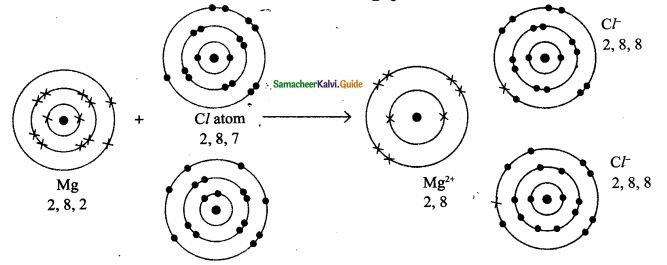(a) Which of the above atom loses electrons to form a cation?
(b) Which of the above atom gain electrons to form an anion?
(c) How many electrons are transferred from Mg to Cl?
(d) Write the name of the anion formed.
(e) Which noble gas configuration do these ions resemble?
(f) Write the electronic configuration of Mg2+ & Cl
(a) Magnesium atom loses electrons to form a cation.
(b) Chlorine atom gains 1 electron to from anion.
(c) Two electrons are transferred from Mg – atom to 2 Cl – atoms (each Cl – atom gains 1e from Mg – atom).
(d) Chloride anion (Cl )
(e) Mg2+ ion resembles noble gas configuration of Neon Cl ion resembles noble gas configuration of Argon.
(f) Electronic configuration of Mg2+ is 2, 8. and Electronic configuration of Cl is 2, 8, 8.Question 5.
What is covalent bond?
Bond which is formed between atoms by the mutual sharing of electrons is known as a covalent bond.

Question 6.
Name of the following:
(a) An element which obtains the noble gas configuration of neon by losing three electrons.
(b) An element which gains two electrons to obtain the noble gas configuration of Neon.
(a) Aluminium (Al → Al3+ + 3e)
(b) Oxygen atom (O + 2e → 02-)

Question 7.
Identify the following reactions as oxidation/reduction/redox reaction.
(a) Zn + CuSO4 → Cu + ZnSO4
(b) CuO+H2 → Cu+H2O
(c) 2Mg +O2  → 2MgO
(a) Redox reaction
(b) Reduction
(e) OxidationQuestion 8.
What are oxidising agents? Give an example.
Substances which have the ability to oxidise other substances are called oxidizing agents. These are also called electron acceptors because they remove electrons from other substances. ’
Example:Question 9.
What are reducing agents? Give examples.
Substances which have the ability to reduce other substances are called reducing agents. These are also called electron donors because they donate electrons to other substances.
Example: NaBH4, LiAlH4 and metals like Palladium, Platinum.

Question 10.
What are redox reactions? Give examples.
Both the oxidation and reduction occurs in the same reaction simultaneously is known as a redox reaction. If one reactant gets oxidised, the other gets reduced. Such reactions are called oxidation-reduction reactions or redox reactions.
Ex. 1 : 2 PbO + C → 2 Pb + CO2
Ex. 2 : Zn + CuSO → Cu + ZnSO4Question 11.
Define (a) oxidation (b) reduction reactions : Give examples.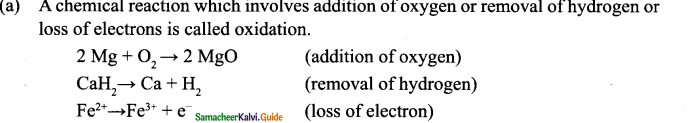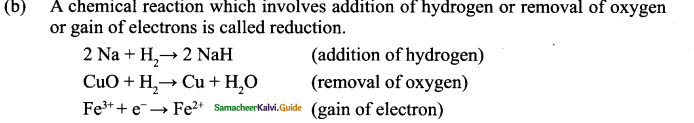Question 12.
What is rancidity?
The oxidation reaction in food materials that were left open for a long period is responsible for spoiling of food. This is called rancidity.Question 13.
Define oxidation number.
Oxidation number of an element is defined as the formal charge which an atom of that element appears to have when electrons are counted.

Question 14.
Identify the type of bond in $$\mathrm{NH}_{4}^{+}$$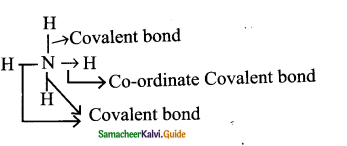VII. To interpret:

Question 1.
Ionic bond is also called electrostatic bond.
In ionic bond formation the bond is formed between the oppositely charged ions and these ions come closer to each other due to electrostatic force of attraction. So ionic bond is also called an electrostatic bond.

Question 2.
Ionic compounds are crystalline solids at room temperature.
Ionic compounds are formed because of the strong electrostatic force between cations and anions which are arranged in a well-defined geometrical pattern. Thus ionic compounds are crystalline solids at room temperature.Question 3.
Covalent compounds have a low melting point.
In covalent compounds, atoms are held by a weak force of attraction. When heat is applied, the molecules are readily pulled out and get free movement.

VIII. Assertion and Reason type questions :

Question 1.
Statement (A) : Ionic compounds do not conduct electricity in a solid-state.
Reason (B) : The ions in ionic compounds are tightly held together by a strong electrostatic force of attraction and they can not move freely.
(a) B explains A
(b) B does not explain A
(c) B is wrong A
(d) A is right B is wrong
(a) B explains AQuestion 2.
Statement (A) : Covalent compounds are bad conductors of electricity.
Reason (B) : Covalent compounds contain charged particles (ions)
a) B explains A
b) B does not explain A
c) Both A & B are right
d) Both A & B are wrong
(b) B does not explain A
Reason: Since covalent compounds do not have charged particles (ions), they are bad conductors of electricity.

IX. Find the oxidation number of the elements in the following compounds.

(1) Zn in ZnSO4
(2) Ca in CaH2
(3) Mg in MgO
(4) N in NH3
(5) A1 in AlCl3
(1) ZnSO4
1(Zn) + 1 (S) + 4 (0) = 0
x + 1(+6) + 4(-2) = 0
x + 6 – 8 = 0
x = + 2
Oxidation number of Zn in ZnSO4 is +2

(2) CaH2
1(Ca) + 2 (H) = 0
x + 2 (-1) = 0
x -2 = 0
x = + 2
Oxidation number of Ca in CaH2 is +2

(3) MgO
1(Mg) + 1 (O) = 0
x + 1 (-2) = 0
x -2 = 0
x = + 2
Oxidation number of Mg in MgO is +2

(4) NH3
1(N) + 3 (H) = 0
x + 3 (-1) = 0
x – 3 = 0
x = +3
Oxidation number of N in NH3 is +3
(5) AlCl3
1(Al) + 3(Cl) = 0
x + 3 (-1) = 0
x – 3 = 0
x = + 3
Oxidation number of Al in AlCl3 is +3X. Complete the following table:

Question 1.

 Atoms Atomic number Electron distribution a) O 8 – b) N – – c) – 17 – d) – – 2,8,2

 Atoms Atomic number Electron distribution a) O 8 2,6 b) N 7 2,5 c) Cl 17 2,8,7 d) Mg 12 2,8,2Question 2.

 a) Dativebond – b) CaH2 → Ca+H2 – c) – Reduction d) – Redox reaction

 a) Dativebond Co-ordinate covalent bond b) CaH2 → Ca+H2 Oxidation c) Fe3+ + e– →Fe2+ Reduction d) both Oxidation of Reduction takes place simultaneously Redox reaction

XI. To Match:

 Name of compound Type of compound a) Sodium chloride ? b) ? co-ordinate covalent compound c) Methane ? d) Fluorine molecule ?

 Name of compound Type of compound a) Sodium chloride Ionic compound b) NH3 → BF3 co-ordinate covalent compound c) Methane Covalent compound d) Fluorine molecule Covalent compoundQuestion 1.
Explain the ionic bond formation in sodium chloride with electron distribution diagram.
Formation of Sodium Chloride (NaCl)

(i) The atomic number of Sodium is 11 and its electronic configuration is 2, 8, 1. It has one electron excess to the nearest stable electronic configuration of a noble gas – Neon.

(ii) So sodium has a tendency to lose one electron from its outermost shell and acquire a stable electronic configuration forming sodium cation (Na+).

(iii) The atomic number of chlorine is 17 and its electronic configuration is 2, 8, 7. It has one electron less to The nearest stable electronic configuration of a noble gas – Argon.

(iv) So chlorine has a tendency to gain one electron to acquire a stable electronic
configuration forming chloride anion (Cl)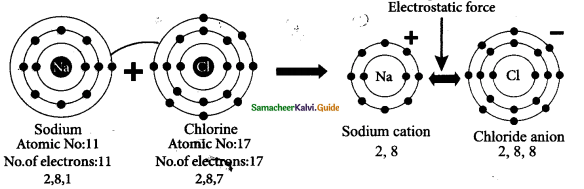(v) When an atom of sodium combines with an atom of chlorine, an electron is transferred from the sodium atom to chlorine atom forming sodium chloride molecule. Thus both the atoms achieve stable octet electronic configuration.

Question 2.
Explain the covalent bond formation in the following molecules.
(a) Chlorine
(b) Nitrogen
(c) Hydrogen
(d) Oxygen
(a) Chlorine
Chlorine molecule is formed by two chlorine atoms. Each chlorine atom has seven valence electrons (2,8,7). These two atoms achieve a stable completely filled electronic configuration (octet) by sharing a pair of electrons.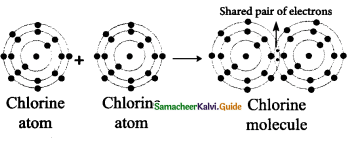(b) Nitrogen
Nitrogen molecule is formed by two nitrogen atoms. Each nitrogen atom has five valence electrons (2, 5). These two atoms achieve a stable completely filled electronic configuration (octet) by sharing three pair of electrons. Hence a triple bond is formed in between the two atoms.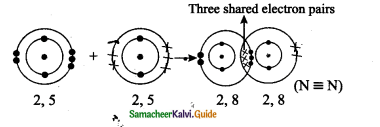(c)Hydrogen
Hydrogen molecule is formed by two hydrogen atoms. Both hydrogen atoms contributes one electron each to the shared pair and both atoms acquire stable completely filled electronic configuration.(d) Oxygen
Oxygen molecule is formed by two oxygen atoms. Each oxygen atom has six valence electrons (2,6). These two atoms achieve a stable electronic configuration (octet) by sharing two pair of electrons. Hence a double bond is formed in between
the two atoms.Question 3.
Explain the co-ordinate covalent bond formation in between NH3 → BF3 molecules.
The ammonia molecule gives a lone pair of electrons to boron trifluoride (BF3) molecule which is electron deficient. Thus a coordinate covalent bond is formed between NH3 (donor molecule) and BF3 (acceptor molecule) and is represented by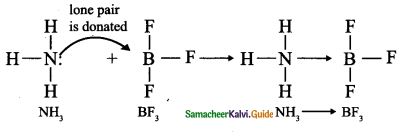Question 4.
Write notes on the characteristics of covalent compounds.
a. Physical state: Depending on the force of attraction between covalent molecules the bond may be weaker or stronger. Thus covalent compounds exist in gaseous, liquid, and solid forms. Eg. Oxygen-gas; Water-liquid: Diamond-solid.

b. Electrical conductivity: Covalent compounds do not contain charged particles (ions), so they are bad conductors of electricity.

c. Melting point: Except few covalent compounds (Diamond, Silicon carbide), they have relatively low melting points compared to Ionic compounds.

d. Solubility: Covalent compounds are readily soluble in non-polar solvents like benzene (C6H6), carbon tetrachloride (CCl4). They are insoluble in polar solvents like water.

e. Hardness and brittleness: Covalent compounds are neither hard nor brittle. But they are soft and waxy.

f. Reactions: Covalent compounds undergo molecular reactions in solutions and these reactions are slow.Question 5.
Write notes on the characteristics of ionic compounds.